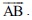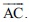# User Forum

Subject :IMO    Class : Class 6

Following are the steps of construction of an angle of 120°. Identify the wrong step.
(i) Draw a ray(ii) With A as centre and a convenient radius, draw an arc to intersectat X.
(iii) With X as the centre, draw an arc with the same radius to intersect the above arc at C.
(iv) With C as centre, again draw an arc to intersect the first arc at D.
(v) Draw ray∠CAB is the required angle of measure 120°

A(iii) and (v) only
B(iv) only
C(i) and (iv) only
D(v) only

ACCORDING TO ME ANSWER IS B